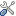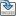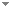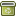# A computable version of Banach’s Inverse Mapping Theorem

Annals of Pure and Applied Logic 157 (2-3):85-96 (2009)

 Abstract Given a program of a linear bounded and bijective operator T, does there exist a program for the inverse operator T−1? And if this is the case, does there exist a general algorithm to transfer a program of T into a program of T−1? This is the inversion problem for computable linear operators on Banach spaces in its non-uniform and uniform formulation, respectively. We study this problem from the point of view of computable analysis which is the Turing machine based theory of computability on Euclidean space and other topological spaces. Using a computable version of Banach’s Inverse Mapping Theorem we can answer the first question positively. Hence, the non-uniform version of the inversion problem is solvable, while a topological argument shows that the uniform version is not. Thus, we are in the striking situation that any computable linear operator has a computable inverse while there exists no general algorithmic procedure to transfer a program of the operator into a program of its inverse. As a consequence, the computable version of Banach’s Inverse Mapping Theorem is a powerful tool which can be used to produce highly non-constructive existence proofs of algorithms. We apply this method to prove that a certain initial value problem admits a computable solution. As a preparation of Banach’s Inverse Mapping Theorem we also study the Open Mapping Theorem and we show that the uniform versions of both theorems are limit computable, which means that they are effectively -measurable with respect to the effective Borel hierarchy Keywords No keywords specified (fix it) Categories Science, Logic, and Mathematics (categorize this paper) DOI 10.1016/j.apal.2008.09.002 OptionsEdit this recordMark as duplicateExport citationFind it on ScholarRequest removal from indexRevision history

PhilArchive copy

Upload a copy of this paper     Check publisher's policy     Papers currently archived: 71,355

Setup an account with your affiliations in order to access resources via your University's proxy server
Configure custom proxy (use this if your affiliation does not provide a proxy)

## References found in this work BETA

On Computable Numbers, with an Application to the N Tscheidungsproblem.Alan Turing - 1936 - Proceedings of the London Mathematical Society 42 (1):230-265.
Effective Borel Measurability and Reducibility of Functions.Vasco Brattka - 2005 - Mathematical Logic Quarterly 51 (1):19-44.
Borel Complexity and Computability of the Hahn–Banach Theorem.Vasco Brattka - 2008 - Archive for Mathematical Logic 46 (7-8):547-564.
Quelques Procédés de Définition En Topologie Récursive.Daniel Lacombe - 1959 - In A. Heyting (ed.), Journal of Symbolic Logic. Amsterdam: North-Holland Pub. Co.. pp. 129--158.

## Similar books and articles

Borel Complexity and Computability of the Hahn–Banach Theorem.Vasco Brattka - 2008 - Archive for Mathematical Logic 46 (7-8):547-564.
How Incomputable Is the Separable Hahn-Banach Theorem?Guido Gherardi & Alberto Marcone - 2009 - Notre Dame Journal of Formal Logic 50 (4):393-425.
A Definitive Constructive Open Mapping Theorem?Douglas Bridges & Hajime Ishihara - 1998 - Mathematical Logic Quarterly 44 (4):545-552.
A Constructive Version of the Spectral Mapping Theorem.Douglas Bridges & Robin Havea - 2001 - Mathematical Logic Quarterly 47 (3):299-304.
Continuity and Nondiscontinuity in Constructive Mathematics.Hajime Ishihara - 1991 - Journal of Symbolic Logic 56 (4):1349-1354.
An Example Related to Gregory’s Theorem.J. Johnson, J. F. Knight, V. Ocasio & S. VanDenDriessche - 2013 - Archive for Mathematical Logic 52 (3-4):419-434.
A Uniformly Computable Implicit Function Theorem.Timothy H. McNicholl - 2008 - Mathematical Logic Quarterly 54 (3):272-279.